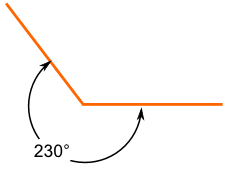# what is meaning of  reflex angle

Dear Students!Best!

• 6

an angle described between 180 degrees and 360 degrees

• -5this is a reflex angle.It is more than 180 but less than 360

• -1

## Reflex Angle

The angle which measures greater than 180o and less than 360o is known as reflex angle. In the figure the angle ABC is a reflex angle.The example of the reflex angle is declaring the more than 180 degree. The following example is declaring reflex angle of 230 degree and 210 degree.The reflex angle is bigger than the half turn. The common angles are no need to calculate the angle accurately with a protractor. The following example is representing the reflex angle.The parts of the reflex angle have two parts. For vertex is the first part. For arms is the second part. The following example is declaring the parts of the reflex angle.The reflex angle is commonly called as the one type of the angle. There are many types of the angles are used. The reflex angle is more than 180 degree. There connect the two straight is called as the vertex. The vertex is singular form. The vertices are the plural form.

If you are satisfied do give thumbs up.

• 13

An angle which is more than 180° but less than 360°.

• 6

more than 180  drs

• -4

a reflex angle is an angle which is greater than 180 degrees and less than 360 degrees.

• 2
What are you looking for?